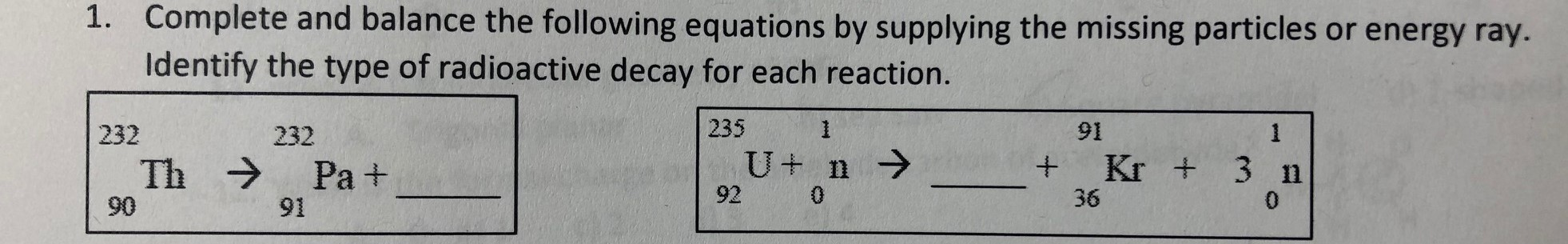# Complete and balance the following equations by supplying the missing particles or energy ray.Identify the type of radioactive decay for each reaction.1.2351911232232U nKr +3 nThPa +9203609091

Question
13 viewshelp_outlineImage TranscriptioncloseComplete and balance the following equations by supplying the missing particles or energy ray. Identify the type of radioactive decay for each reaction. 1. 235 1 91 1 232 232 U n Kr + 3 n Th Pa + 92 0 36 0 90 91 fullscreen
check_circle

Step 1

The reactions involving formation of new nucleus with emission of some radiation along with it from an original nucleus are known as nuclear reactions.

To write a nuclear reaction the chemical equation for the reaction must be balanced that is the sum of atomic number and mass number on both the side should be equal.

Step 2

If protactinium-232 is formed by decay of thorium-232 then to write a balanced nuclear equation following steps should be followed which are given as follows:

1. Write the incomplete reaction showing original nucleus with atomic number and mass number and the particle emitted on the left and right side respectively as follows:
Step 3

2. Determine atomic number and mass number or particle emitted on right side as follows:

Atomic number: The sum of atomic number on both side must be equal in a chemical reaction. Therefore, charge of new particle will be calculated by simply subtracting the atomic number of protactinium (91) from atomic number of thorium (90) as follows:

90 - 91 =...

### Want to see the full answer?

See Solution

#### Want to see this answer and more?

Solutions are written by subject experts who are available 24/7. Questions are typically answered within 1 hour.*

See Solution
*Response times may vary by subject and question.
Tagged in

### Chemical Kinetics